Journal of Integer Sequences, Vol. 13 (2010), Article 10.5.6

## Analogues of Up-down Permutations for Colored Permutations

### Andrew Niedermaier and Jeffrey Remmel Department of Mathematics University of California, San Diego La Jolla, CA 92093-0112 USA

Abstract:

André proved that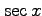is the generating function of all up-down permutations of even length and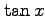is the generating function of all up-down permutation of odd length. There are three equivalent ways to define up-down permutations in the symmetric group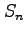. That is, a permutation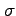in the symmetric groupis an up-down permutation if either (i) the rise set ofconsists of all the odd numbers less than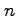, (ii) the descent set ofconsists of all even number less than, or (iii) both (i) and (ii). We consider analogues of André's results for colored permutations of the form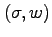where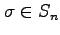and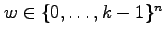under the product order. That is, we define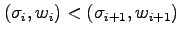if and only if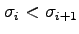and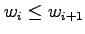. We then say a colored permutationis (I) an up-not up permutation if the rise set ofconsists of all the odd numbers less than, (II) a not down-down permutation if the descent set ofconsists of all the even numbers less than, (III) an up-down permutation if both (I) and (II) hold. For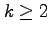, conditions (I), (II), and (III) are pairwise distinct. We find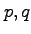-analogues of the generating functions for up-not up, not down-down, and up-down colored permutations.

Full version:  pdf,    dvi,    ps,    latex

(Concerned with sequences A000111 A000182 A122045.)

Received December 19 2009; revised version received May 5 2010. Published in Journal of Integer Sequences, May 5 2010.